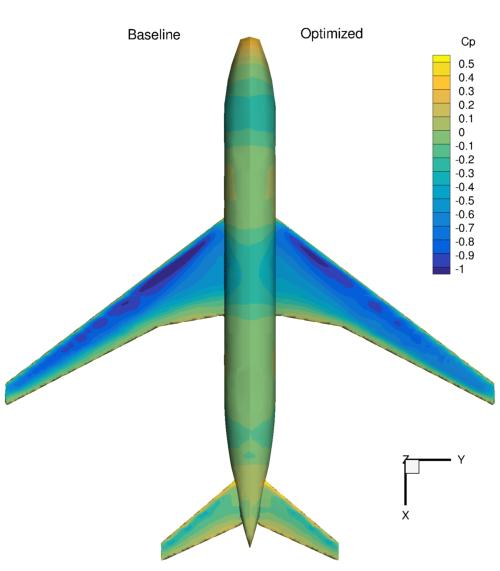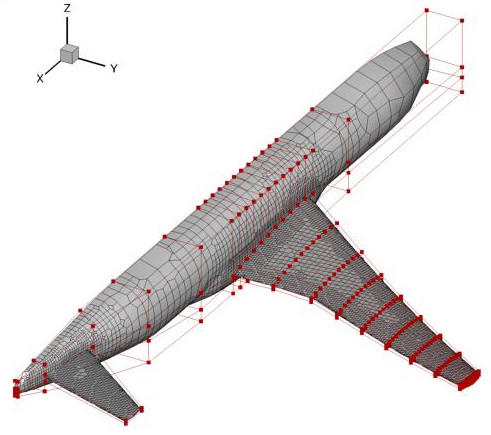# Aircraft wing-body-tail configuration¶

NOTE: Before running this case, please read the instructions in NACA0012 airfoil incompressible to get an overall idea of the DAFoam optimization setup.

This is a trimmed aerodynamic shape optimization case for an aircraft wing-body-tail configuration at transonic conditions. The summary of the case is as follows:

Case: Aircraft aerodynamic optimization
Geometry: CRM wing, body, and tail
Objective function: Drag coefficient
Design variables: 216 FFD points moving in the z direction, 9 wing twists, one tail rotation, one angle of attack
Constraints: Volume, thickness, LE/TE, and lift constraints (total number: 771)
Mach number: 0.85
Reynolds number: 5 million
Mesh cells: 100K

The configuration files are available at Github. To run this case, first source the DAFoam environment (see Tutorials). Then you can go into the run folder and run:

```./Allrun.sh 4
```

The optimization progress will then be written in the log.opt file. NOTE, we recommend running this case on an HPC system using at least 4 CPU cores.

For this case, the optimization converges in 20 steps, see the following figure. The baseline design has C_D=0.05242, C_L=0.5000, C_M=-0.02611 and the optimized design has C_D=0.04997, C_L=0.4999, C_M=0.00015.In this case, we need to use rhoSimpleCDAFoam, a compressible solver that uses the SIMPLEC algorithm. The case setup is similar to the NACA0012 airfoil compressible except that we have more design variables and constraints. The mesh and FFD points are as follows.We use the OpenFOAM’s built-in mesh tool `snappyHexMesh` to generate the unstructured hexa mesh. We use ICEM to generate the body-fitted FFD points. We define two more global design variables: twist and tailTwist:

```def twist(val, geo):
# Set all the twist values
for i in xrange(nTwist):
geo.rot_y['wing'].coef[i+1] = val[i]

# Also set the twist of the root to the SOB twist
geo.rot_y['wing'].coef = val

def tailTwist(val, geo):
# Set one twist angle for the tail
geo.rot_y['tail'].coef[:] = val
```

We then add them into the DVGeo object:

```DVGeo.addGeoDVGlobal('twist', 0*np.zeros(nTwist), twist,lower=lower, upper=upper, scale=0.1)
DVGeo.addGeoDVGlobal('tail', 0*np.zeros(1), tailTwist,lower=-10, upper=10, scale=0.1)
```